# Lightning Potential Index JKg Yair et al 2010

• Slides: 11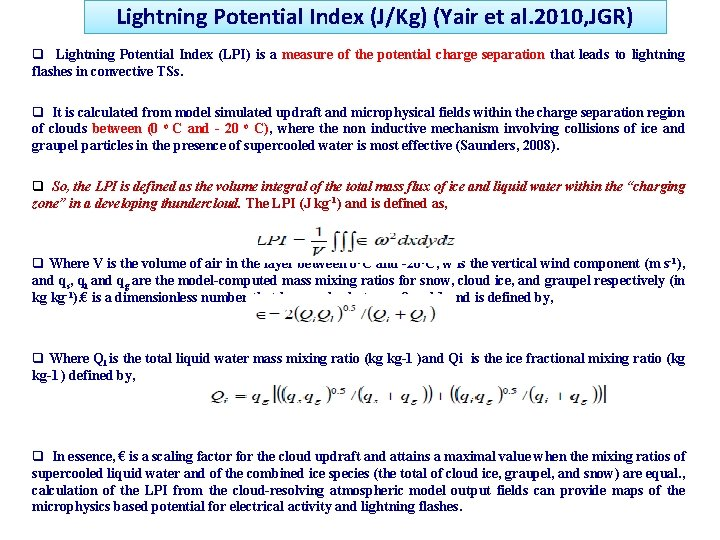Lightning Potential Index (J/Kg) (Yair et al. 2010, JGR) q Lightning Potential Index (LPI) is a measure of the potential charge separation that leads to lightning flashes in convective TSs. q It is calculated from model simulated updraft and microphysical fields within the charge separation region of clouds between (0 o C and - 20 o C), where the non inductive mechanism involving collisions of ice and graupel particles in the presence of supercooled water is most effective (Saunders, 2008). q So, the LPI is defined as the volume integral of the total mass flux of ice and liquid water within the “charging zone” in a developing thundercloud. The LPI (J kg-1) and is defined as, q Where V is the volume of air in the layer between 0 o. C and -20 o. C, w is the vertical wind component (m s-1), and qs, qi and qg are the model-computed mass mixing ratios for snow, cloud ice, and graupel respectively (in kg kg-1). € is a dimensionless number that has a value between 0 and 1 and is defined by, q Where Ql is the total liquid water mass mixing ratio (kg kg-1 )and Qi is the ice fractional mixing ratio (kg kg-1 ) defined by, q In essence, € is a scaling factor for the cloud updraft and attains a maximal value when the mixing ratios of supercooled liquid water and of the combined ice species (the total of cloud ice, graupel, and snow) are equal. , calculation of the LPI from the cloud-resolving atmospheric model output fields can provide maps of the microphysics based potential for electrical activity and lightning flashes.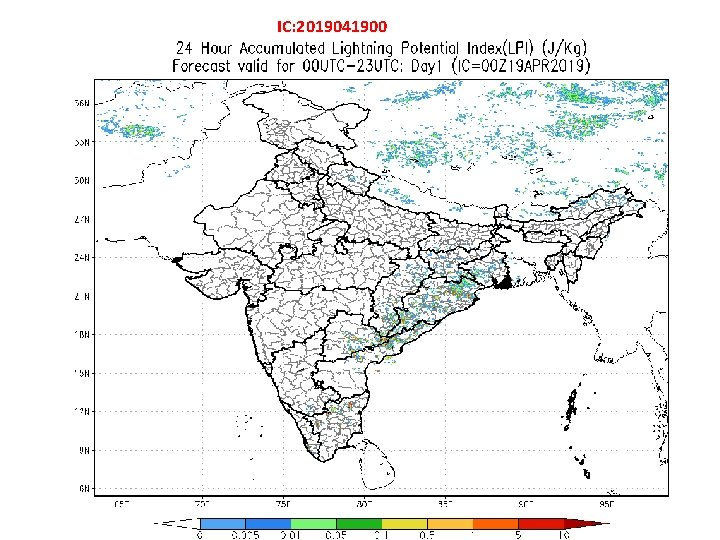IC: 2019041900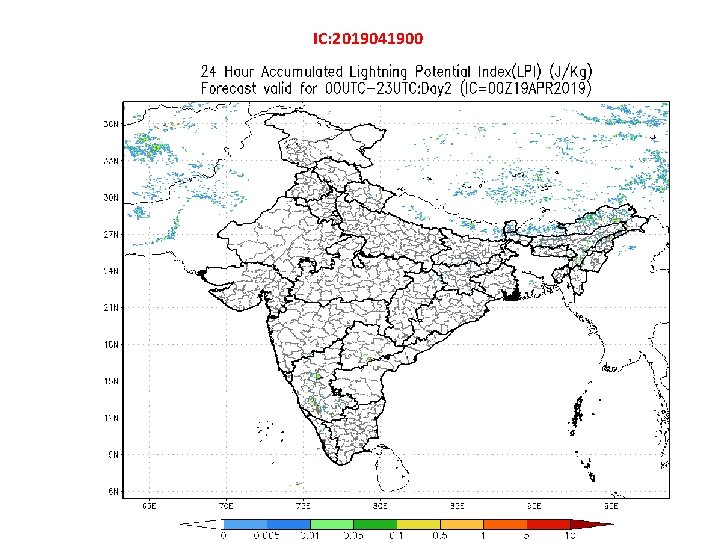IC: 2019041900Day 1 IC: 2019041900Day 2 IC: 2019041900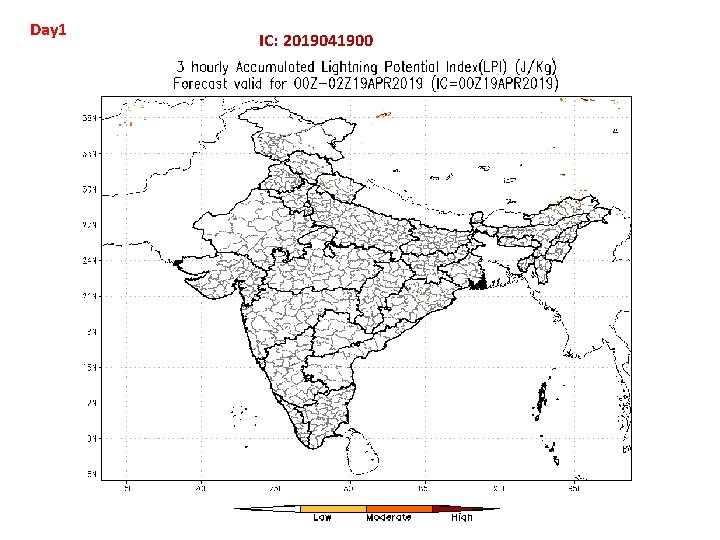Day 1 IC: 2019041900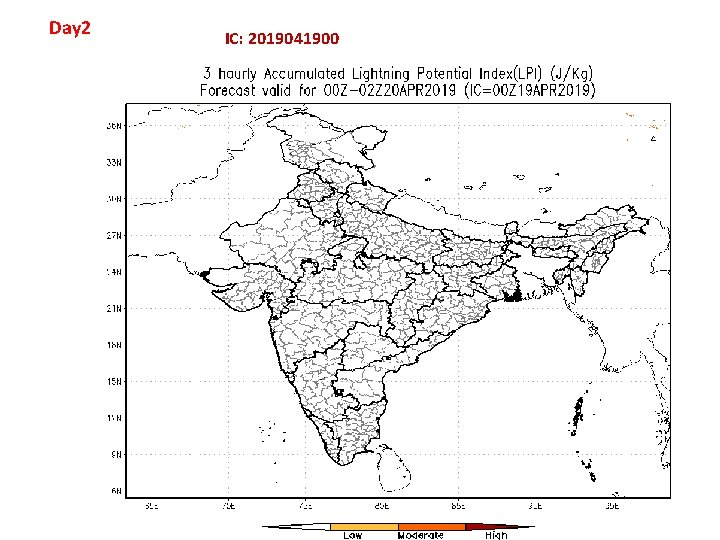Day 2 IC: 2019041900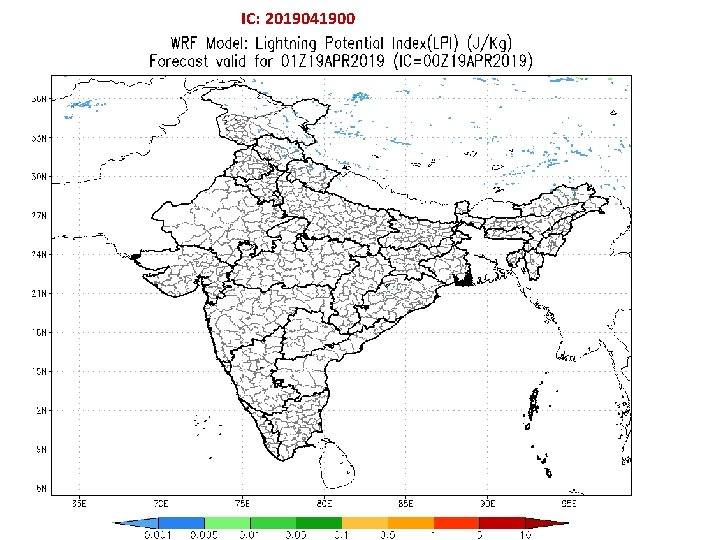IC: 2019041900 Hourly evolution of IMD: WRF (3 km) Derived : Lightning Potential Index (J/kg)IC: 2019041900 Threat Level : LOW: LPI < 0. 001 and >0. 0005 Threat Level : Moderate: LPI : < 0. 01 and > 0. 001 Threat Level : High : LPI > 0. 01IC: 2019041900 SCP: Supercell Composite parameter Hourly evolution of IMD: WRF (3 km) Derived : Supercell Composite Parameter Threat Level : LOW: 3 < SCP < 5 Moderate: 5 < SCP < 7 High : SCP > 7IC: 2019041900 LAYRH : Layer Mean Relative Humidity Hourly evolution of IMD: WRF (3 km) Derived : Layer Mean Relative Humidity Threat Level : LOW: 20 < LAYRH < 40 Moderate: 40 < LAYRH < 60 High : LAYRH > 60